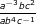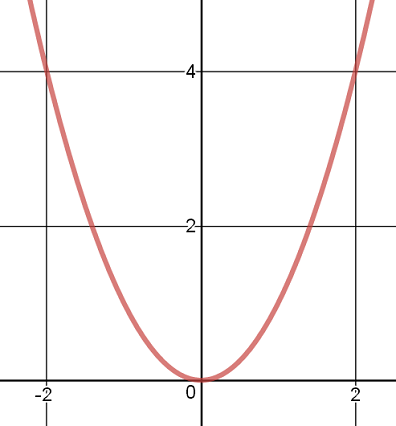# Graphing Functions Flashcards

Graphing Functions Flashcards
1/8 (missed) 0 0
Create Your Account To Continue Studying

As a member, you'll also get unlimited access to over 75,000 lessons in math, English, science, history, and more. Plus, get practice tests, quizzes, and personalized coaching to help you succeed.

Try it risk-free for 30 days. Cancel anytime.
###### Already registered? Login here for access
Write the equation of the line that has a slope of -2 and contains the point (-1, 4).
The equation of the line is y = -2x + 2
Got it

Use the properties of exponents to rewrite the following expression:

a0

a0 = 1
Got it
State the slope of the line that will be graphed by this equation: 4 - y = 2x
The equation in slope-intercept form will be y = -2x + 4. Therefore, the slope of the line is -2.
Got it
Use the properties of polynomials to simplify the expression shown below.The simplified expression is c3/(a4 b3)
Got it

### Ready to move on?

or choose a specific lesson: See all lessons in this chapter
8 cards in set

## Flashcard Content Overview

Do you remember what kind of function creates what kind of graph? Parabolas, Vs, and lines - oh, my! These flashcards will help you practice graphing a few different types of functions: linear, quadratic, and absolute value. You can also review how to use the properties of exponents that apply to multiplication, division, and powers of polynomials.

As you are working through these flashcards, you may find that you want to review some of the functions in greater detail. The following links may help you get the information that you need.

## Studying with Flashcards

If you are reviewing polynomials and graphing functions for an upcoming test, you can use these flashcards to practice some of those concepts. The calculations are all fairly easy, so you should not need a calculator. However, you might want to have some scratch paper handy, to jot down some of the intermediate steps for a few of the problems.

Front
Back
Use the properties of polynomials to simplify the expression shown below.The simplified expression is c3/(a4 b3)
State the slope of the line that will be graphed by this equation: 4 - y = 2x
The equation in slope-intercept form will be y = -2x + 4. Therefore, the slope of the line is -2.

Use the properties of exponents to rewrite the following expression:

a0

a0 = 1
Write the equation of the line that has a slope of -2 and contains the point (-1, 4).
The equation of the line is y = -2x + 2
Origin on the Cartesian plane
The origin on the Cartesian plane is the point (0, 0). It is located where the x- axis and the y-axis cross each other.
Describe the shape of the graph of f(x) = |x| + 2.
The graph of any absolute value function is shaped like a V.
Describe the type of function that would graph as a parabola.
Any polynomial function with x2 in its highest power term will graph as a parabola.
Give the equation of the function shown:The equation is y = x2.

To unlock this flashcard set you must be a Study.com Member.